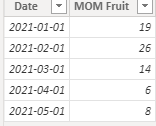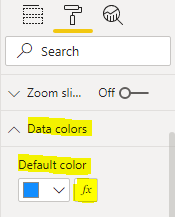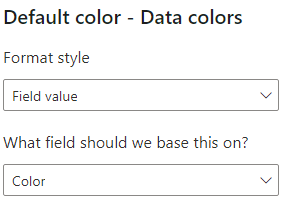cancel
Showing results for
Did you mean:New Member

## Format bar chart colours

Hi

I have a bar chart that needs to show colour changes depending on whether the values have increased or decreased from the previous Month.

For example -

I have a calculated field called MOM Fruit

Jan = 19

Feb = 26

Mar = 14

Apr = 6

May = 8

So, I need Feb to be Red, Mar Green, Apr Green and May Red

Any help would be great

1 ACCEPTED SOLUTIONCommunity Support

I created some data:Here are the steps you can follow：

1. Create measure.

``````Color =
var _current=CALCULATE(SUM('Table'[MOM Fruit]),FILTER(ALL('Table'),MONTH('Table'[Date])=MONTH(MAX('Table'[Date]))))
var _last=CALCULATE(SUM('Table'[MOM Fruit]),FILTER(ALL('Table'),MONTH('Table'[Date])=MONTH(MAX('Table'[Date])-1)))
var _if=
IF(
_last=BLANK(),_current,_last)
var _su=
_current - _if
return
SWITCH(
TRUE(),
_su=0,"blue",
_su>0,"red",
_su<0,"green")``````

2. Click Visual - Format - Data colors -- fx3. Enter the Default color interface.

Format style – Field value

What field should we base this on – [color]4. Result:Best Regards,

Liu Yang

If this post helps, then please consider Accept it as the solution to help the other members find it more quickly

3 REPLIES 3Community Support

I created some data:Here are the steps you can follow：

1. Create measure.

``````Color =
var _current=CALCULATE(SUM('Table'[MOM Fruit]),FILTER(ALL('Table'),MONTH('Table'[Date])=MONTH(MAX('Table'[Date]))))
var _last=CALCULATE(SUM('Table'[MOM Fruit]),FILTER(ALL('Table'),MONTH('Table'[Date])=MONTH(MAX('Table'[Date])-1)))
var _if=
IF(
_last=BLANK(),_current,_last)
var _su=
_current - _if
return
SWITCH(
TRUE(),
_su=0,"blue",
_su>0,"red",
_su<0,"green")``````

2. Click Visual - Format - Data colors -- fx3. Enter the Default color interface.

Format style – Field value

What field should we base this on – [color]4. Result:Best Regards,

Liu Yang

If this post helps, then please consider Accept it as the solution to help the other members find it more quicklySuper User

Hello @kevbrown1980.

Assuming you have a date table, you could create a measure using this pattern:

``````Bar formatting =
VAR PriorMonthMOMFruit =
CALCULATE ( SUM ( 'Table'[MOM Fruit] ), DATEADD ( 'Date'[Date], -1, MONTH ) )
VAR CurrentMonthMOMFruit =
CALCULATE ( SUM ( 'Table'[MOM Fruit] ) )
VAR Result =
IF ( ( CurrentMonthMOMFruit - PriorMonthMOMFruit ) > 0, "Green", "Red" )
RETURN
Result``````

Note that you can replace "Green" and "Red" with hex values if you want to fine-tune the shade of the colour.

Then, select your bar chart, and in the visualization pane, under Data colors, click the "fx" button:On the next screen, under the "Format style" drop-down at the top, choose "Field value".

Then, under "What field should we base this on", choose the measure you created above.

Your results should look something like this:-SteveSuper User

@kevbrown1980 , create a measure like this example and use it conditional formatting using field value option

Colour =
SWITCH(TRUE(),
max('Table'[Month]) = "Feb", "red",
max('Table'[Month]) = "Mar", "Green",

max('Table'[Month]) = "Apr", "Green",

max('Table'[Month]) = "May", "Red",
"green")

How to do conditional formatting by measure and apply it on pie?: https://youtu.be/RqBb5eBf_I4Announcements#### Exclusive opportunity for Women!

Join us for a free, hands-on Microsoft workshop led by women trainers for women where you will learn how to build a Dashboard in a Day!#### Power Platform Conference-Power BI and Fabric Sessions

Join us Oct 1 - 6 in Las Vegas for the Microsoft Power Platform Conference.Top Solution Authors
Top Kudoed Authors
Users online (3,522)